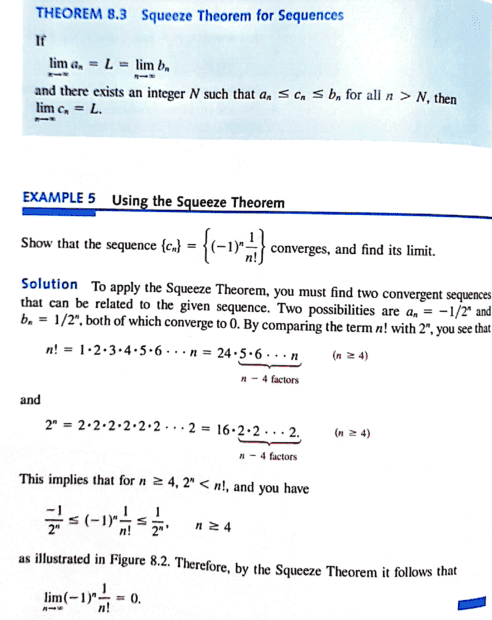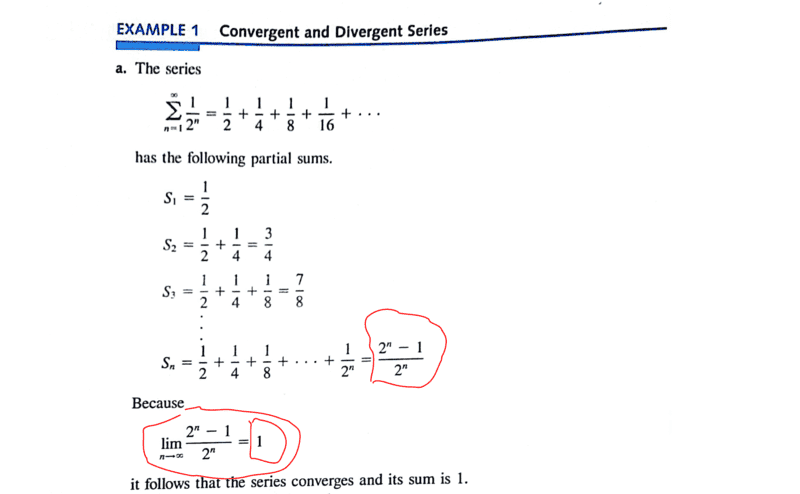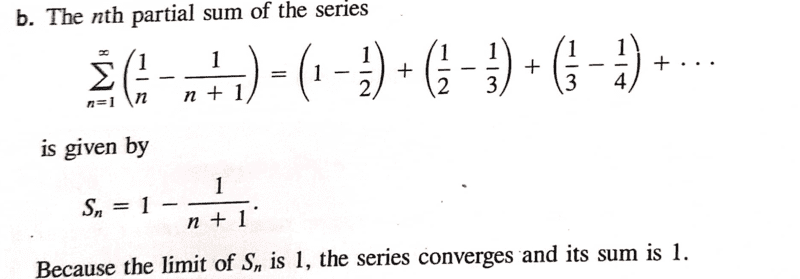# Understanding the squeeze theorem

• I
Gold Member
TL;DR Summary
See attachedThe theorem is pretty clear...out of curiosity i would like to ask...what if we took ##n-3## factors...then the theorem would not be true because we shall have;

##[n!=6⋅4⋅5⋅6 ... n]##

and

##[2^n = 8⋅2⋅2⋅2 ...2]##

What i am trying to ask is at what point do we determine the number of terms to consider? Is there a general rule when using Squeeze Theorem or is it a matter of one using common sense?Of course i can see that for ##n## values greater than or equal to ##4## the theorem would be sufficient.

Mentor
2022 Award
TL;DR Summary: See attached

What i am trying to ask is at what point do we determine the number of terms to consider?
We have ##\dfrac{-1}{2^n}\leq \dfrac{(-1)^n}{n!}\leq \dfrac{1}{2^n}.## In order to squeeze something, we need ##\dfrac{1}{n!}<\dfrac{1}{2^n}## which is equivalent to ##2^n<n!## and this isn't true for ##n\leq 3,## hence ##n>3.##

•malawi_glenn and chwala
Gold Member
We have ##\dfrac{-1}{2^n}\leq \dfrac{(-1)^n}{n!}\leq \dfrac{1}{2^n}.## In order to squeeze something, we need ##\dfrac{1}{n!}<\dfrac{1}{2^n}## which is equivalent to ##2^n<n!## and this isn't true for ##n\leq 3,## hence ##n>3.##
That is exactly what I am saying, ...I agree... then it's just a matter of logical thinking ( checking the comparison and so forth) ...what about sequences that may have alternating terms? would the theorem still apply? I may need to explore further. Thanks @fresh_42 .

Mentor
2022 Award
That is exactly what I am saying, ...I agree... then it's just a matter of logical thinking ( checking the comparison and so forth) ...what about sequences that may have alternating terms? would the theorem still apply? I may need to explore further. Thanks @fresh_42 .
Be careful. If ##a_n\leq b_n \leq c_n## - alternating or not - and ##L:=\lim_{n \to \infty}a_n## and ##M:=\lim_{n \to \infty}c_n## then ##L=M## implies ##\lim_{n \to \infty}b_n=L. ## The important condition is ##L=M,## not convergence. If ##L\neq M## then we cannot make any conclusions without further information like e.g. monotony.

•malawi_glenn and chwala
Gold Member
Be careful. If ##a_n\leq b_n \leq c_n## - alternating or not - and ##L:=\lim_{n \to \infty}a_n## and ##M:=\lim_{n \to \infty}c_n## then ##L=M## implies ##\lim_{n \to \infty}b_n=L. ## The important condition is ##L=M,## not convergence. If ##L\neq M## then we cannot make any conclusions without further information like e.g. monotony.
Nice, i see that it does not matter whether a sequence is alternating or not as the Absolute Value theorem will apply. Cheers mate.

Gold Member
Still on this, instead of using a similar sequence...can't we just use limits straightaway? i am asking this in reference to another example;

For this case; we may have

##\lim_{n→∞} \left[(-1)^n \dfrac {1}{n!}\right] =0##

My next example is on determining the divergence or convergence of the sequence;

##b_n = (-1)^n \dfrac{n}{n+1}##

it follows that;

##\lim_{n→∞} \left[(-1)^n \dfrac {n}{n+1}\right] =\left[\lim_{n→∞} (-1)^n\right]⋅\left[\lim_{n→∞} \dfrac {n}{n+1}\right]=∞⋅1=∞##

thus sequence diverges.

i hope i am doing it right...cheers

Last edited:
Gold Member
* i do not want to post a new thread as the questions are related...

Also for

##c_n = \dfrac{ \ln (n^2)}{n}##

here i used the L 'Hopital's rule i.e

##\lim_{x→∞} \dfrac {\ln (x^2)}{x}= \lim_{x→∞} \left[\dfrac{2}{x}\right]=0##

The sequence converges to ##0##.

Last edited:
Gold Member
...on the highlighted i hope my reasoning is correct any further insight is welcome...refreshing a little bit...On the first highlighted part to arrive there, they made use of;

##Sn= \dfrac{a(1-r^n)}{1-r}= \dfrac{0.5(1-0.5^n)}{1-0.5}=1- \dfrac{1^n}{2^n}= \dfrac{2^n -1 }{2^n}##

on the second highlighted they made use of L'Hopital's rule;

##\lim_{x→∞} \dfrac {2^x-1}{2^x}= \lim_{x→∞} \left[\dfrac{2^x \ln 2}{2^x \ln 2}\right]=1##

Another approach;

Also ...the series is geometric, therefore ##r=\dfrac{1}{2}## because ##0< |r|< 1##, the series converges and its sum is

##S = \dfrac{a}{1-r} = \dfrac {0.5}{1-0.5} = 1##

Last edited:
Gold Member
This series;can also be expressed as one fraction i.e

##S_n= \dfrac{1}{n(n+1)}## ...the partial sums converge to ##1##. We have ##[0.5, 0.666, 0.75, 0.8, ...)##

##\lim_{n→∞} \dfrac{1}{n(n+1)}=\lim_{n→∞}\left[ \dfrac{A}{n} + \dfrac{B}{1+n}\right] ##

With

##A=1##

##A+B=0, ⇒B=-1##

then the results given will be realized...this is just but a different way of me looking at the problem...of course the text approach is straightforward no dispute there.

Mentor
2022 Award
Still on this, instead of using a similar sequence...can't we just use limits straightaway? i am asking this in reference to another example;

For this case; we may have

##\lim_{n→∞} \left[(-1)^n \dfrac {1}{n!}\right] =0##

My next example is on determining the divergence or convergence of the sequence;

##b_n = (-1)^n \dfrac{n}{n+1}##

it follows that;

##\lim_{n→∞} \left[(-1)^n \dfrac {n}{n+1}\right] =\left[\lim_{n→∞} (-1)^n\right]⋅\left[\lim_{n→∞} \dfrac {n}{n+1}\right]=∞⋅1=∞##

thus sequence diverges.

i hope i am doing it right...cheers
You cannot write it that way. ##\lim_{n \to \infty}(-1)^n## does not exists, and even less is it ##\infty .## To show divergence, you could e.g. simply show that ##|a_n-a_{n-1}|>0.5.## Limit formulas do not help in such a case.

Mentor
2022 Award
* i do not want to post a new thread as the questions are related...

Also for

##c_n = \dfrac{ \ln (n^2)}{n}##

here i used the L 'Hopital's rule i.e

##\lim_{x→∞} \dfrac {\ln (x^2)}{x}= \lim_{x→∞} \left[\dfrac{2}{x}\right]=0##

The sequence converges to ##0##.
You need to justify why you can transition from discrete to continuous. Also, how exactly did you apply L'Hôpital? Wikipedia's version is
$$\lim_{x \to x_0}\dfrac{f'(x)}{g'(x)}=c \Longrightarrow \lim_{x \to x_0}\dfrac{f(x)}{g(x)}=c$$
Yours seem to be different?!?

Mentor
2022 Award
...on the highlighted i hope my reasoning is correct any further insight is welcome...refreshing a little bit...

View attachment 316322

On the first highlighted part to arrive there, they made use of;

##Sn= \dfrac{a(1-r^n)}{1-r}= \dfrac{0.5(1-0.5^n)}{1-0.5}=1- \dfrac{1^n}{2^n}= \dfrac{2^n -1 }{2^n}##

on the second highlighted they made use of L'Hopital's rule;

##\lim_{x→∞} \dfrac {2^x-1}{2^x}= \lim_{x→∞} \left[\dfrac{2^x \ln 2}{2^x \ln 2}\right]=1##

Another approach;

Also ...the series is geometric, therefore ##r=\dfrac{1}{2}## because ##0< |r|< 1##, the series converges and its sum is

##S = \dfrac{a}{1-r} = \dfrac {0.5}{1-0.5} = 1##
Same problem with L'Hôpital as before. See above.

Mentor
2022 Award
Sorry, but this thread is getting a mess by discussing four posts in parallel.

•